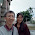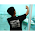2013年3月20日 星期三

你懂 JIT compiler 了嗎？

JVM 當中負責這些最佳化的部份稱之為 Just in Time（JIT）compiler。 它利用了一個有趣的程式特性： 大多數時間都花在少數的程式碼上，幾乎所有程式都是這樣。 有別於將你的程式碼整個 compile， JIT（Java HotSpot VM）用 interpreter 立即執行程式、 然後在執行時分析你的程式碼，找出那些 hot spot。 Then it focuses the attention of a global native-code optimizer on the hot spots. 透過避免 compile 極少執行的程式碼， Java HotSpot compiler 可以把正更多注意力放在程式中影響效能的關鍵部份。 這代表你的 compile 時間整體來說不會增加。 在程式執行中會持續動態監測 hot spot， 所以它會根據你的 application 使用方式來即時調整效能。

JIT 用了很多技術來達到效能優勢，像是去除沒用的 code、 跳過邊界值檢查、移除多餘的載入、inline method...... 等等>

1. 還沒作最佳化的程式碼：

`````` class Calculator {
Wrapper wrapper;
public void calculate() {
y = wrapper.get();
z = wrapper.get();
sum = y + z;
}
}

class Wrapper {
final int value;
final int get() {
return value;
}
}``````
2. 最佳化過後的程式碼：

`````` class Calculator {
Wrapper wrapper;
public void calculate() {
y = wrapper.value;
sum = y + y;
}
}

class Wrapper {
final int value;
final int get() {
return value;
}
}``````

1. 還沒最佳化的程式碼。在還沒被判定成 hot spot 時，程式碼會這樣執行：

`````` class Calculator {
Wrapper wrapper;
public void calculate() {
y = wrapper.get();
z = wrapper.get();
sum = y + z;
}
}``````
2. inline method：用 `b.value` 取代 `wrapper.get()`， 不透過函數呼叫而直接存取 `wrapper.value` 來減少延遲。

`````` class Calculator {
Wrapper wrapper;
public void calculate() {
y = wrapper.value;
z = wrapper.value;
sum = y + z;
}
}``````
3. 移除多餘的載入：用 `z = y` 取代 `z = wrapper.value`， 所以只存取區域變數而不是 `wrapper.value` 來減少延遲。

`````` class Calculator {
Wrapper wrapper;
public void calculate() {
y = wrapper.value;
z = y;
sum = y + z;
}
}``````
4. copy propagation：用 `y = y` 取代 `z = y`， 沒有必要再用一個變數 `z`，因為 `z``y` 會是相等的。

`````` class Calculator {
Wrapper wrapper;
public void calculate() {
y = wrapper.value;
y = y;
sum = y + y;
}
}``````
5. 消除不用的程式碼：`y = y` 是不必要的，可以消滅掉。

`````` class Calculator {
Wrapper wrapper;
public void calculate() {
y = wrapper.value;
sum = y + y;
}
}``````

2 則留言:

1.5.消除不用的程式碼：y = y 是不必要的，可以消滅掉。

class Calculator {
Wrapper wrapper;
public void calculate() {
y = wrapper.value;
sum = y + y;
}
}

上頭sum = y + y;是不是打錯了？應該是sum = y + z;對嗎？

2.不，沒有錯，倒讓我發現是第四步有個 typo。第四步的 sum = y + z; 應該是 sum = y + y;

也就是說 JIT 在第四步就會發現 z 根本就是沒用的變數，所以會直接用 y 取代 z。